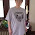## Sunday, May 21, 2017

### Jared Kushner

Jared Kushner in Simple Gematria Equals: 134
Tisha B'Av = 7+18+8+19+26 + 25 + 26+5 = 134 (Reverse Ordinal)
Tisha Bav in Jewish Gematria Equals: 911
Jared Kushner in Jewish Gematria Equals: 1123
Moscow in Jewish Gematria Equals: 1123
Moscow in Simple Gematria Equals: 88
Trump in Simple Gematria Equals: 88
Tisha B'Av = 7+5+6+6+1 + 2 + 1+5 = 33 (Septenary)

Kushner Companies = 66+231+190+36+105+15+171 + 6+120+91+136+1+105+45+15+190 = 1523 (Trigonal)
Prime Yes - 241st
July 4th USA 241st birthday

Jared Corey Kushner (born January 10, 1981
Kushner birthdate
1+10+19+81=111
Mark Of Beast in Simple Gematria Equals: 111
Mark Of Beast in English Gematria Equals: 666
1+1+0+1+9+8+1=21=Saturn

1.Kushner equals 666 in standard Hebrew gematria

1.2.3.